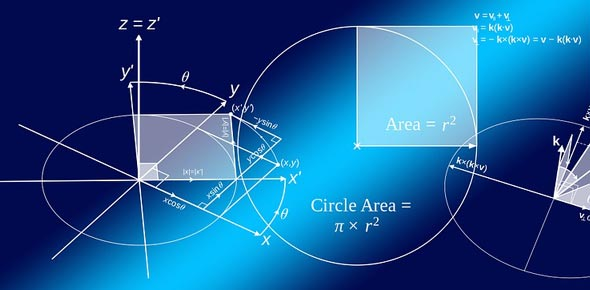# Mathematical Functions (Sum, Sub, Mul, Div, Min, Max)

10 Questions | Attempts: 296
ShareSettings• 1.
What is the correct formula of sum?
• A.

=sum(F9;F1)

• B.

=sum(F9:F1)

• C.

-sum(f9,F1)

• 2.
What does SUMIFS function do?
• A.

Add up cell values based on a condition

• B.

Add all the numbers in a range of cells

• C.

Returns a subtotal in a list or database

• D.

All of Above

• 3.
Cell A5 shows a figure of 645123.875. Which of the following functions will display to the number is thousand?
• A.

=Round(A5,-3)

• B.

=Round(A5,-2)

• C.

=Round(A5,-1)

• 4.
What does SUMIF function do?
• A.

Adds up cell values based on a condition

• B.

Adds all the numbers in a range of cells

• C.

Returns a subtotal in a list or database

• D.

All of Above

• 5.
In Excel, what is the correct way to multiply the numbers?
• A.

=15*3

• B.

15*3

• C.

None of Above

• 6.
A Text can be sum with the sum funciton.
• A.

True

• B.

False

• 7.
Which is the correct way to take out the percentage?
• A.

=450*550/100

• B.

=550/450*100

• C.

=450/550*100

• 8.
(range , criteria, [sum_range]) In which formula  these aurguments work?
• A.

Sum

• B.

Sumif

• C.

Countif

• 9.
what will be the answer of =2+3*5
• A.

30

• B.

25

• C.

17

• 10.
"<>" Why we use this operator
• A.

This is not an operator

• B.

When we want to compare the Greater than and Less Then value together

• C.

Not Equal to

## Related TopicsBack to top
×

Wait!
Here's an interesting quiz for you.